# Math practice

Math Practice is a totally free program which is user friendly, colorful and easy to use. Note that you MUST have the JRE (Java Runtime Environment) installed for this program to work. The JRE is also totally free.

Math Practice has three basic sections. There are worksheets, basic math and questions. Basic math is perfect to learn how to quickly do calculations in your head. Once you have mastered this you can move on to worksheets which can be any type of question as they can be manually set. Math Practice comes with more than 20 worksheets which deal with fractions, decimals, exponents, roots and more! There is also a detailed help section on how to do the sums and on how to go about setting your own worksheet. The last section is there as a tool to memorize facts: you set yourself questions and give the answer and then try to answer the questions you have set.

Questions are automatically generated and then marked and afterwards you get a detailed breakdown of your results. There

## Related software (5)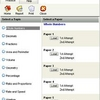## Higher Mathematics Quiz

For students aged 10 - 12 in Primary 4 - 6. There are over 2000 challenging Maths quizzes and problem sums to practise on. Topics include Addition, Subtraction, Multiplication, Di ...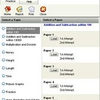## Mathematics Quiz

For students aged 7 - 9 in Primary 1 - 3. There are over 1500 challenging Maths quizzes and problem sums to practise on. Topics include Addition, Subtraction, Multiplication, Divi ...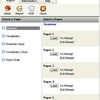## Primary 1 Test Papers

Primary 1 English and Maths test papers. 1500 challenging quizzes to practice. Questions are modelled closely to primary education curriculum. All test papers comes with model ans ...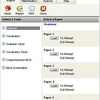## Primary 3 Test Papers

Primary 3 English, Maths & Science test papers. 2500 challenging quizzes to practice. Questions are modelled closely to primary education curriculum. All test papers comes with mo ...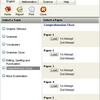## Primary 6 Test Papers

Primary 6 English, Maths & Science test papers. 3000 challenging quizzes to practice. Questions are modelled closely to primary education curriculum. All test papers comes with mo ...